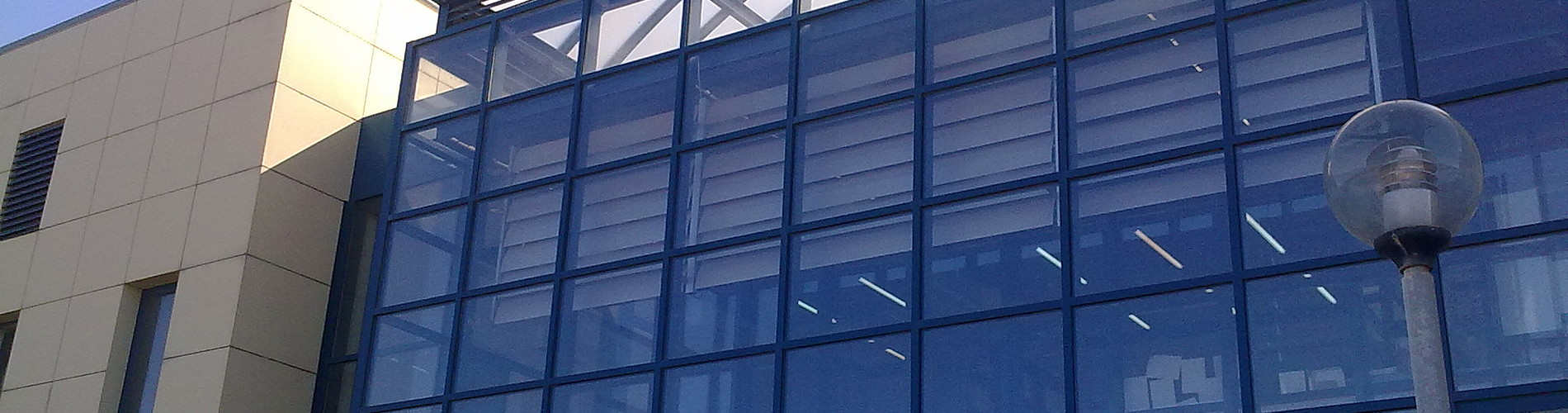School of Chemical and Environmental Engineering

Now offering two distinct diplomas: Chemical Engineering and Environmental Engineering

# Ordinary differential equations

1. COURSE INFORMATION:

 School Environmental Engineering Course Level Undergraduate Course ID MATH 203 Semester 3rd Course Category Required Course Modules Instruction Hours per Week ECTS Lectures 3 Th=3, E=0, L=0 5 Course Type Scientific area Prerequisites Instruction/Exam Language Greek The course is offered to Erasmus students Yes Course URL https//www.eclass.tuc.gr/courses/MHPER310/   (in Greek)

2. LEARNING OUTCOMES

 Learning Outcomes In many problems / systems of everyday life we ​care about  the change of a quantity (variable) of our interest w.r.t. time (rate of change). In order to model (mathematically) and solve these problems we use differential equations. The role of d.e. is therefore a protagonist in many scientific fields, especially in engineering. The purpose of this undergraduate course is to introduce basic concepts and techniques to students in order to be able to solve ordinary differential equations. Furthermore, applications of d.e. are being presented in basic problems/fields of Mechanics, Electromagnetism, Environment and so on. The aim of the course is also, to give the students the ability to use differential equations to model natural phenomena / situations, to be able to solve such equations and to interpret the solutions of the equations. The course is addressed to the sophomore students of the School / Department of Environmental Engineering of the Technical University of Crete. General Competencies/Skills Work autonomously Review, analyse and synthesise data and information, with the use of necessary technologies) Team work

3. COURSE SYLLABUS

 Introduction. 1st  and 2nd order d.e.: separation of variables, homogenous, exact, Bernoulli, Ricati, Euler, integrating factors. Newton's differential equation and applications in engineering problems. Linear independence, Wronskian. Linear differential equations with constant coefficients. Laplace transform method. Applications in Engineering and Electricity 1st order systems with constant coefficients.. Power series method

4. INSTRUCTION and LEARNING METHODS - ASSESSMENT

 Lecture Method Direct (face to face) Use of Information and Communication Technology Specialized software, Power point presentations, E-class support Instruction Organisation Activity Workload per Semester (hours) - Lectures 39 - Review exercises 6 -Software applications 6 - Autonomous study 74 Course Total 125 Assessment Method Ι. Written final examination (100%). Questions of theoretical knowledge. Theoretical problems to be resolved. II. “Bonus” exercises (10% in addition to the final exam). OR I. Midterm and final exam (100%=50%+50%) II.“Bonus” exercises (10% in addition to the project grade).